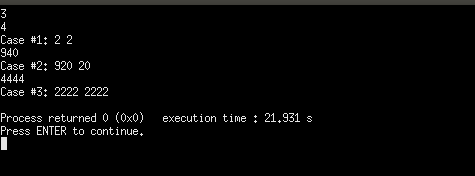Q:

# Google CodeJam 2019 | Foregone Solution

Someone just won the Code Jam lottery, and we owe them N jamcoins! However, when we tried to print out an oversized check, we encountered a problem. The value of N, which is an integer, includes at least one digit that is a 4... and the 4 key on the keyboard of our oversized check printer is broken.

Fortunately, we have a workaround: we will send our winner two checks for positive integer amounts A and B, such that neither A nor B contains any digit that is a 4, and A + B = N. Please help us find any pair of values A and B that satisfy these conditions.

Input

The first line of the input gives the number of test cases, TT test cases follow; each consists of one line with an integer N.

Output

For each test case, output one line containing Case #x: A B, where x is the test case number (starting from 1), and A and B are positive integers as described above.

It is guaranteed that at least one solution exists. If there are multiple solutions, you may output any one of them.

Sample

 Input Output 349404444 Case #1: 2 2Case #2: 852 88Case #3: 667 3777

Reference: Foregone Solution

Explanation:

In Sample Case #1, notice that A and B can be the same. The only other possible answers are 1 3 and 3 1.

Note: Before going to solution, please try it by yourself.

Short description of solution approach

For any number N, let say, 45234, we can write it as 45234 + 00000 that is N = A+B, where A = 45234 and B = 00000. But, according to given condition there should not be any '4' in A or B. So, whenever we encounter '4' in A we can write it as 2 and at the same position at B we can put 2.

Example:

```    Input: 45234
Step 1:
45234	← A
+00000  ← B
--------------------------------
45234   ← N
---------------------------------
Step 2:
25232   ← A
+20002  ← B
--------------------------
45234	← N
---------------------------```

Algorithm

```    Step1: Take input N (as a string)
Step2: Take an array B of size N and initialize all values to 0
Step3: for(i=0;i<N.length();i++)
Step3.1: if(N[i]=='4')
B[i]='2';
N[i]=2;
Step4: print N (that is A) and B
```

Explanation

C++ implementation:

``````#include <bits/stdc++.h>
#define ll long long int;

using namespace std;
int main()
{
int T,k=1;

cin>>T;

while(T--)
{
string N;
cin>>N;
int i,len=0;
len=N.length();         //Length of N
//Taking vector B of size len (Size of N)
//and initialize all values to 0
vector<int>B(len,0);
for(i=0;i<len;i++)
{
//Checking if N[i] is 4 or not
if(N[i]=='4')
{
N[i]='2';       //If 4 replace it by 2
B[i]=2;         //Also replace B[i] by 2
}
}

int ind=-1;
/*If there is any leading 0 in B then we should not print that.
So, moving the index to very first non zero value of B*/
for(i=0;i<len;i++)
{
//checking if there is any more leading 0 or not
if(B[i]!=0)
{
ind=i;
break;
}
}

//printing the value of A
cout<<"Case #"<<k<<": "<<N<<" ";
//printing the value of B without leading 0
for(i=ind;i<len;i++)
cout<<B[i];
cout<<"\n";
//k is for printing the Case Number
k++;
}

return 0;
}``````

Output